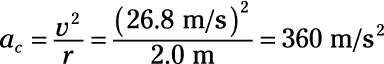##### Physics I Workbook For Dummies with Online Practice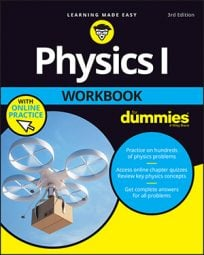In order to keep an object going around in a circle, that object must be pulled toward the center of the circle. Take a look at the Moon circling Earth in the following figure. The Moon is accelerated toward Earth along a radius from Earth to the Moon.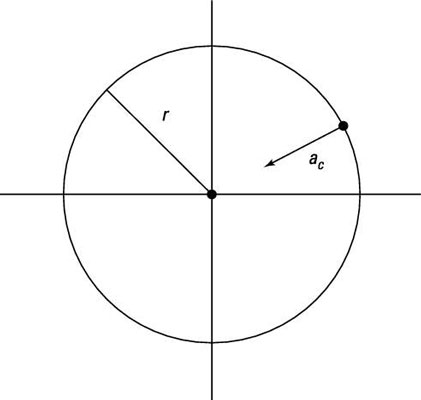The acceleration needed to keep an object (here, it's the Moon) going around in a circle is called the centripetal acceleration, and it's always perpendicular to the object's travel. The centripetal acceleration points toward the center of the circle.

If you're given the linear speed of the object going in a circle and the radius of the circle, you can calculate the centripetal acceleration, ac, like this: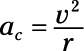This equation gives you the acceleration needed to keep an object going around in a circle. You can think of this acceleration as continually bending the direction the object is going in so it will keep going in a circle.

The linear velocity v of an object going around in a circle is directly related to the angular velocity omega and the radius of the circle: v = omega x r. So you can also write this equation as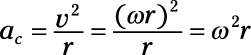## Sample question

1. Given that the Moon goes around Earth about every 27.3 days and that its distance from the center of Earth is 3.85 x 108 m, what is the moon's centripetal acceleration?

The correct answer is 2.7 x 10–3 m/s2.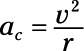2. Find the speed of the moon. It goes 2ðr in 27.3 days, so convert 27.3 to seconds: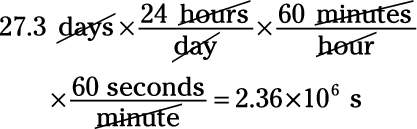3. Therefore, the speed of the moon is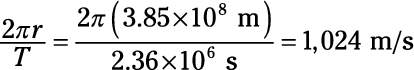4. Plug in the numbers: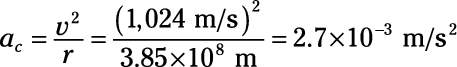## Practice questions

1. The tips of a helicopter's blades are moving at 300 m/s and have a radius of 7.0 m. What is the centripetal acceleration of those tips?

2. Your ball on a string is revolving around in a circle. If it's going 60 mph at a radius of 2.0 m, what is its centripetal acceleration?

Following are answers to the practice questions:

1. 1.3 x 104 m/s2

1. Use this equation:2. Plug in the numbers: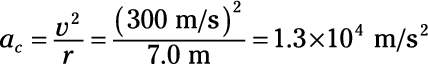2. 360 m/s2

1. Use this equation:2. Convert the 60 miles/hour speed to m/s: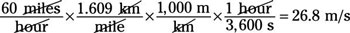3. Plug in the numbers: